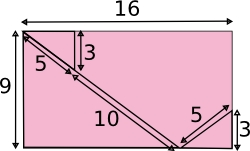#### You may also like### Square Pegs

Which is a better fit, a square peg in a round hole or a round peg in a square hole?### The Old Goats

A rectangular field has two posts with a ring on top of each post. There are two quarrelsome goats and plenty of ropes which you can tie to their collars. How can you secure them so they can't fight each other but can reach every corner of the field?### Isosceles

Prove that a triangle with sides of length 5, 5 and 6 has the same area as a triangle with sides of length 5, 5 and 8. Find other pairs of non-congruent isosceles triangles which have equal areas.

# Rectangle Dissection

##### Age 11 to 14 ShortChallenge Level

The 16 by 9 rectangle is cut as shown.If the pieces are rearranged to form a square, what is the perimeter of the square?

If you liked this problem, here is an NRICH task which challenges you to use similar mathematical ideas.

This problem is taken from the UKMT Mathematical Challenges.
You can find more short problems, arranged by curriculum topic, in our short problems collection.